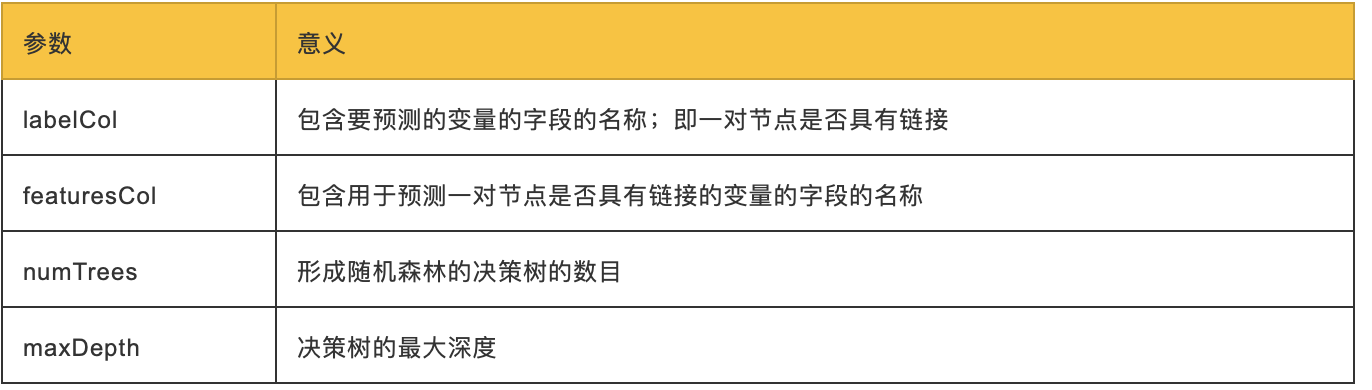## 均衡和拆分数据

``````def down_sample(df):
copy = df.copy()
zero = Counter(copy.label.values)
un = Counter(copy.label.values)
n = zero - un
copy = copy.drop(copy[copy.label == 0].sample(n=n, random_state=1).index)
return copy.sample(frac=1)
``````

``````train_existing_links = graph.run("""
MATCH (author:Author)-[:CO_AUTHOR_EARLY]->(other:Author)
RETURN id(author) AS node1, id(other) AS node2, 1 AS label
""").to_data_frame()
MATCH (author:Author)
WHERE (author)-[:CO_AUTHOR_EARLY]-()
MATCH (author)-[:CO_AUTHOR_EARLY*2..3]-(other)
WHERE not((author)-[:CO_AUTHOR_EARLY]-(other))
RETURN id(author) AS node1, id(other) AS node2, 0 AS label
""").to_data_frame()
training_df['label'] = training_df['label'].astype('category')
training_df = down_sample(training_df)
training_data = spark.createDataFrame(training_df)
``````

``````training_data.show(n=5)
+-------+-------+-------+
| node1 | node2 | label |
+-------+-------+-------+
| 10019 | 28091 | 1     |
| 10170 | 51476 | 1     |
| 10259 | 17140 | 0     |
| 10259 | 26047 | 1     |
| 10293 | 71349 | 1     |
+-------+-------+-------+
``````

``````training_data.groupby("label").count().show()
``````

``````+-------+-------+
| label | count |
+-------+-------+
| 0     | 81096 |
| 1     | 81096 |
+-------+-------+
``````

``````test_existing_links = graph.run("""
MATCH (author:Author)-[:CO_AUTHOR_LATE]->(other:Author)
RETURN id(author) AS node1, id(other) AS node2, 1 AS label
""").to_data_frame()
MATCH (author:Author)
WHERE (author)-[:CO_AUTHOR_LATE]-()
MATCH (author)-[:CO_AUTHOR*2..3]-(other)
WHERE not((author)-[:CO_AUTHOR]-(other))
RETURN id(author) AS node1, id(other) AS node2, 0 AS label
""").to_data_frame()
test_df['label'] = test_df['label'].astype('category')
test_df = down_sample(test_df)
test_data = spark.createDataFrame(test_df)
``````

``````test_data.groupby("label").count().show()
``````

``````+-------+-------+
| label | count |
+-------+-------+
| 0     | 74128 |
| 1     | 74128 |
+-------+-------+
``````

## 如何预测缺少的链接

• 更多共同作者
• 作者之间潜在的三元关系
• 有更多任意类型关系的作者
• 同一社区的作者（这里的社区指的是community detection算法中的社区，并非物理上的实际的社区，下同）
• 同一个更紧密社区的作者

#### 创建机器学习的管道

``````def create_pipeline(fields):
assembler = VectorAssembler(inputCols=fields, outputCol="features")
rf = RandomForestClassifier(labelCol="label", featuresCol="features",
numTrees=30, maxDepth=10)
return Pipeline(stages=[assembler, rf])
``````

RandomForestClassifier使用以下参数：我们根据实验选择决策树的数量和深度。我们可以考虑超参数，比如可以调整以优化性能的算法设置。最佳超参数通常很难提前确定，而调整模型通常需要一些尝试和错误。

#### 预测链接：基本的图特征

• Common authors：查找两个作者之间的潜在三角形数。这抓住了这样一个观点，即两个有共同作者的作者将来可能会被介绍和合作。
• Preferential attachment（择优附着）通过将每对作者的合著者数量相乘，为每对作者生成一个分数。直觉是已经和其他人合作的作者更有可能开展合作。
• Total union of neighbors：查找每个作者的合著者总数，减去重复项。 在Neo4j中，我们可以使用Cypher查询计算这些值。以下函数将计算训练集的这些度量：
``````def apply_graphy_training_features(data):
query = """
UNWIND \$pairs AS pair
MATCH (p1) WHERE id(p1) = pair.node1
MATCH (p2) WHERE id(p2) = pair.node2
RETURN pair.node1 AS node1,
pair.node2 AS node2,
size([(p1)-[:CO_AUTHOR_EARLY]-(a)-
[:CO_AUTHOR_EARLY]-(p2) | a]) AS commonAuthors,
size((p1)-[:CO_AUTHOR_EARLY]-()) * size((p2)-
[:CO_AUTHOR_EARLY]-()) AS prefAttachment,
size(apoc.coll.toSet(
[(p1)-[:CO_AUTHOR_EARLY]-(a) | id(a)] +
[(p2)-[:CO_AUTHOR_EARLY]-(a) | id(a)]
)) AS totalNeighbors
"""
pairs = [{"node1": row["node1"], "node2": row["node2"]}
for row in data.collect()]
features = spark.createDataFrame(graph.run(query,
{"pairs": pairs}).to_data_frame())
return data.join(features, ["node1", "node2”])
``````

``````def apply_graphy_test_features(data):
query = """
UNWIND \$pairs AS pair
MATCH (p1) WHERE id(p1) = pair.node1
MATCH (p2) WHERE id(p2) = pair.node2
RETURN pair.node1 AS node1,
pair.node2 AS node2,
size([(p1)-[:CO_AUTHOR]-(a)-[:CO_AUTHOR]-(p2) | a]) AS commonAuthors,
size((p1)-[:CO_AUTHOR]-()) * size((p2)-[:CO_AUTHOR]-())
AS prefAttachment,
size(apoc.coll.toSet(
[(p1)-[:CO_AUTHOR]-(a) | id(a)] + [(p2)-[:CO_AUTHOR]-(a) | id(a)]
)) AS totalNeighbors
"""
pairs = [{"node1": row["node1"], "node2": row["node2"]}
for row in data.collect()]
features = spark.createDataFrame(graph.run(query,
{"pairs": pairs}).to_data_frame())
return data.join(features, ["node1", "node2”])
``````

``````training_data = apply_graphy_training_features(training_data)
test_data = apply_graphy_test_features(test_data)
``````

``````plt.style.use('fivethirtyeight')
fig, axs = plt.subplots(1, 2, figsize=(18, 7), sharey=True)
charts = [(1, "have collaborated"), (0, "haven't collaborated”)]
for index, chart in enumerate(charts):
label, title = chart
filtered = training_data.filter(training_data["label"] == label)
common_authors = filtered.toPandas()["commonAuthors"]
histogram = common_authors.value_counts().sort_index()
histogram /= float(histogram.sum())
histogram.plot(kind="bar", x='Common Authors', color="darkblue",
ax=axs[index], title=f"Authors who {title} (label={label})")
axs[index].xaxis.set_label_text("Common Authors")
plt.tight_layout()
plt.show()
``````

``````def train_model(fields, training_data):
pipeline = create_pipeline(fields)
model = pipeline.fit(training_data)
return model
``````

``````basic_model = train_model(["commonAuthors"], training_data)
``````

``````eval_df = spark.createDataFrame(
[(0,), (1,), (2,), (10,), (100,)],
['commonAuthors'])
(basic_model.transform(eval_df)
.select("commonAuthors", "probability", "prediction")
.show(truncate=False))
``````

``````+---------------+------------------------------------------+------------+
| commonAuthors | probability                              | prediction |
+---------------+------------------------------------------+------------+
| 0             | [0.7540494940434322,0.24595050595656787] | 0.0        |
| 1             | [0.7540494940434322,0.24595050595656787] | 0.0        |
| 2             | [0.0536835525078107,0.9463164474921892]  | 1.0        |
| 10            | [0.0536835525078107,0.9463164474921892]  | 1.0        |
+---------------+------------------------------------------+------------+
``````